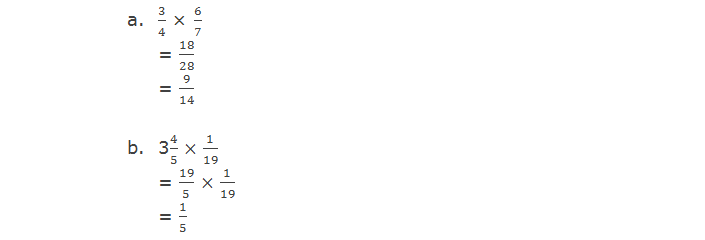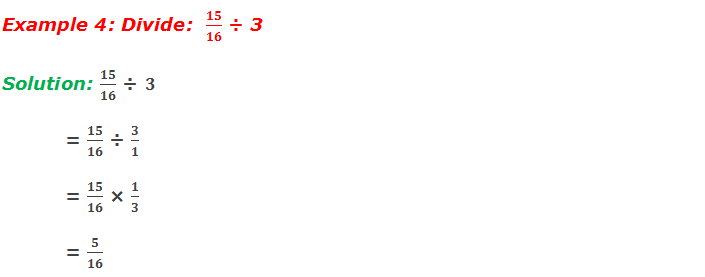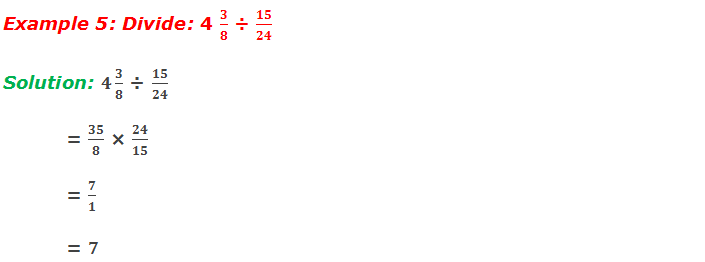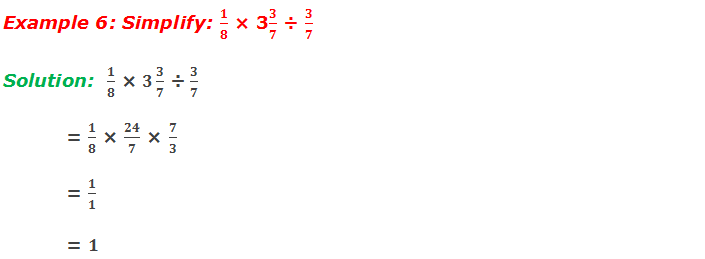# Multiplication and Division of Fractions

## Multiplication of Fractions

### Multiplication of a fraction by a whole number

********************

10 Math Problems officially announces the release of Quick Math Solver, an Android App on the Google Play Store for students around the world.

********************

In multiplication of a fraction by a whole number we use the process of repeated addition.

For example,

In short method, we multiply the numerator by a whole number and keep the denominator as it is.

For example,

Multiplication of a fraction by a fraction

In multiplication of a fraction by a fraction, we divide the same whole object into two fractions. The common shaded part is the product of the fractions.

For example,

In a short method, we follow the following steps to multiply the fractions:

a. If there is a mixed fraction, change it to improper fraction.

b. Numerators and denominators should be cancelled by common factors.

c. Numerators and denominators are multiplied.

d. If the product is an improper fraction, change it to a mixed fraction.

For example,## Division of Fractions

### Division of a fraction by a fraction

In division of a fraction by a fraction or when we divide a fraction by another fraction, we multiply the dividend fraction with the reciprocal of its divisor fraction.

The reciprocal of a fraction is the fraction obtained by interchanging its numerator and denominator.

For example:

So, we simply change the divide (÷) sign into multiply (×) sign and interchange the numerator and denominator of the divisor. Then, we multiply the fractions.

For example,

## Workout ExamplesYou can comment your questions or problems regarding the multiplication and division of fractions here.